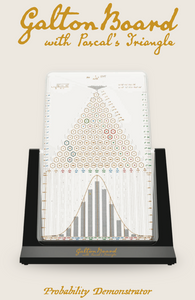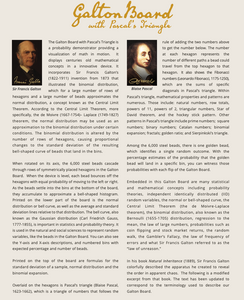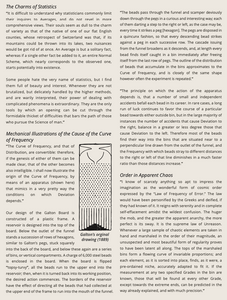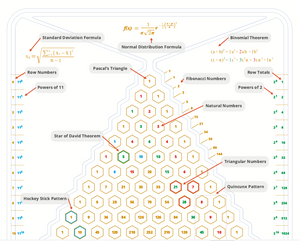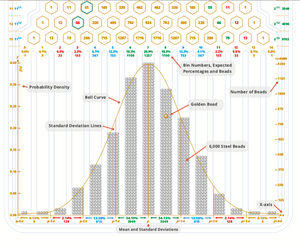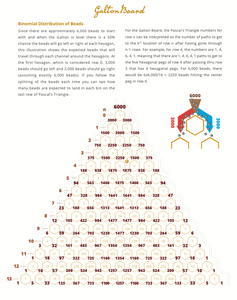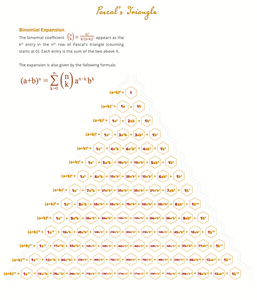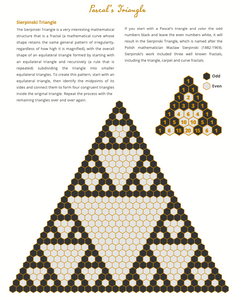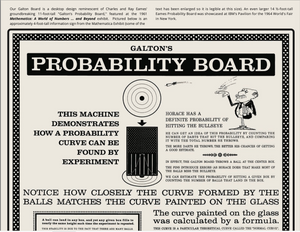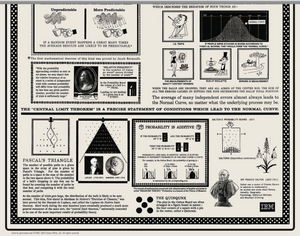# Galton Board with Pascal's Triangle

Regular price \$89.95 Sale

The Galton Board with Pascal’s Triangle is a 12" x 8.5" probability demonstrator providing a visualization of math in motion. It displays centuries old mathematical concepts in a innovative device. It incorporates Sir Francis Galton’s (1822-1911) invention from 1873 that illustrated the binomial distribution, which for a large number of rows of hexagons and a large number of beads approximates the normal distribution, a concept known as the Central Limit Theorem. According to the Central Limit Theorem, more specifically, the de Moivre (1667-1754)– Laplace (1749-1827) theorem, the normal distribution may be used as an approximation to the binomial distribution under certain conditions. The binomial distribution is altered by the number of rows of hexagons, causing proportional changes to the standard deviation of the resulting bell-shaped curve of beads that land in the bins.

When rotated on its axis, the 6,000 steel beads cascade through rows of symmetrically placed hexagons in the Galton Board. When the device is level, each bead bounces off the hexagons with equal probability of moving to the left or right. As the beads settle into the bins at the bottom of the board, they accumulate to approximate a bell-shaped histogram. Printed on the lower part of the board is the normal distribution or bell curve, as well as the average and standard deviation lines relative to that distribution. The bell curve, also known as the Gaussian distribution (Carl Friedrich Gauss, 1777-1855), is important in statistics and probability theory. It is used in the natural and social sciences to represent random variables, like the beads in the Galton Board. You can also see the Y-axis and X-axis descriptions, and numbered bins with expected percentage and number of beads.

Printed on the top of the board are formulas for the standard deviation of a sample, normal distribution and the binomial expansion.

Overlaid on the hexagons is Pascal’s triangle (Blaise Pascal, 1623-1662), which is a triangle of numbers that follows the rule of adding the two numbers above to get the number below. The number at each hexagon represents the number of different paths a bead could travel from the top hexagon to that hexagon. It also shows the Fibonacci numbers (Leonardo Fibonacci, 1175-1250), which are the sums of specific diagonals in Pascal’s triangle. Within

Pascal’s triangle, mathematical properties and patterns are numerous. Those include: natural numbers, row totals, powers of 11, powers of 2, triangular numbers, Star of David theorem, and the hockey stick pattern. Other patterns in Pascal’s triangle include prime numbers; square numbers; binary numbers; Catalan numbers; binomial expansion; fractals; golden ratio; and Sierpinkski’s triangle.

Among the 6,000 steel beads, there is one golden bead, which identifies a single random outcome. With the percentage estimates of the probability that the goldenbead will land in a specific bin, you can witness those probabilities with each flip of the Galton Board.